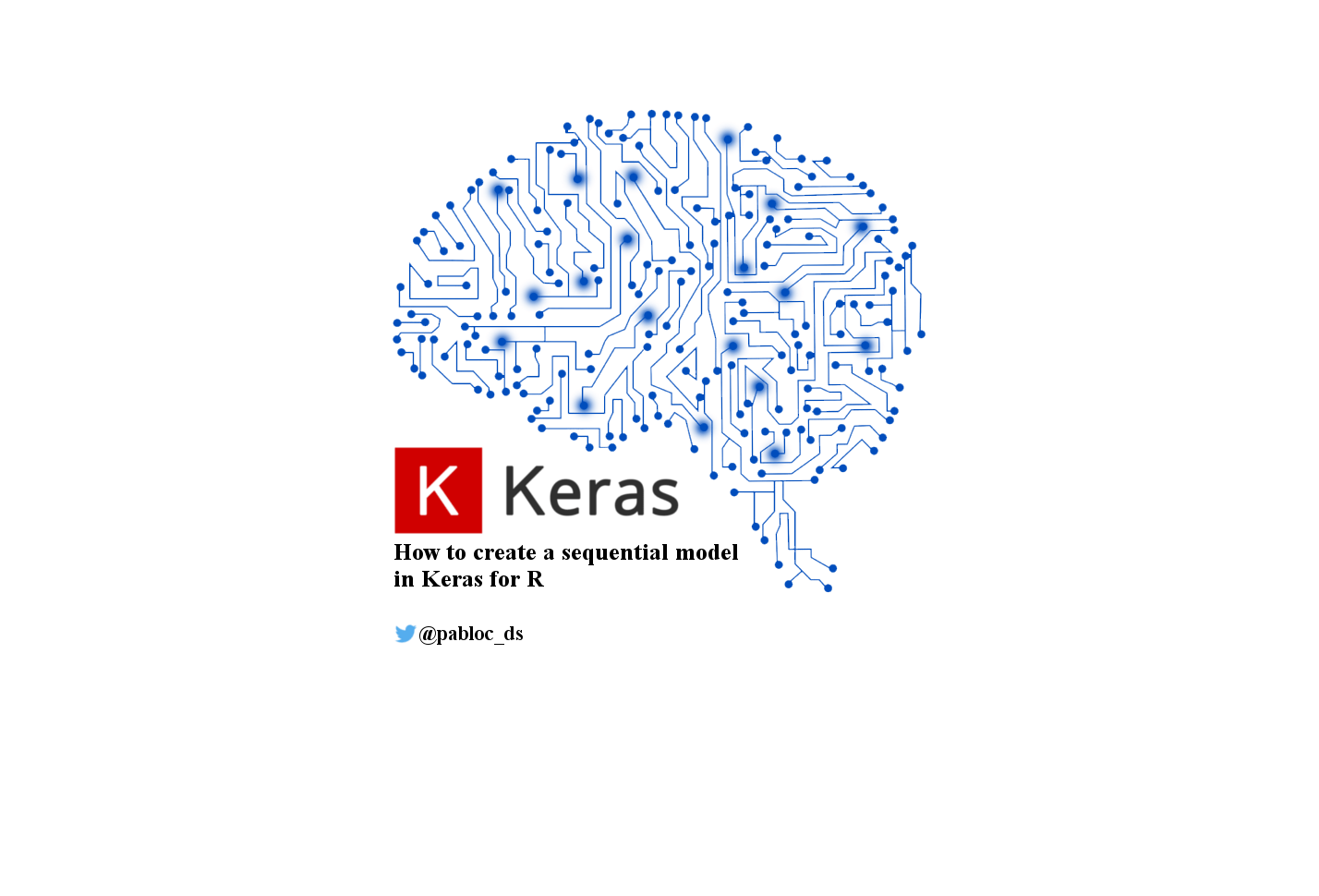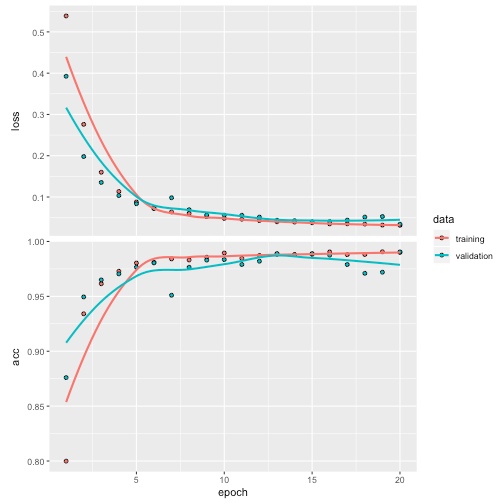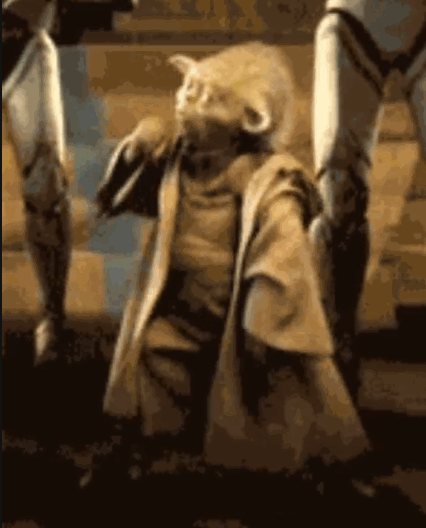[This article was first published on R - Data Science Heroes Blog, and kindly contributed to R-bloggers]. (You can report issue about the content on this page here)
Want to share your content on R-bloggers? click here if you have a blog, or here if you don't.tl;dr: This tutorial will introduce the Deep Learning classification task with Keras. We will particularly focus on the shape of the arrays, which is one of the most common pitfalls.

The topics we’ll cover are:

• How to do one-hot encoding
• Choosing the input and output shape/dimensions in the layers
• How to train the model
• How to evaluate the model (training vs. test)

You can find the complete code of this tutorial on Github ????## Creating the data

The input data will be 10000 rows and three columns coming from the uniform distribution.

The model will recognize that the sum of the three numbers is above a threshold of `1.5`, otherwise it will be 0.

```# Input: 10000 rows and 3 columns of uniform distribution
x_data=matrix(data=runif(30000), nrow=10000, ncol=3)

# Output
y_data=ifelse(rowSums(x_data) > 1.5, 1, 0)

##            [,1]      [,2]      [,3]
## [1,] 0.49224109 0.6096538 0.7855929
## [2,] 0.56710301 0.8707570 0.3428543
## [3,] 0.08246592 0.7504529 0.6979251
## [4,] 0.79197829 0.6330420 0.7307403
## [5,] 0.65170373 0.9052915 0.1395298
## [6,] 0.23479406 0.2611791 0.2780037

##  1 1 1 1 1 0
```

```# install.packages("keras")
library(keras)
library(tidyverse)
```

### One-hot encoding in Keras

One of the key points in Deep Learning is to understand the dimensions of the vector, matrices and/or arrays that the model needs. I found that these are the types supported by Keras.

In Python’s words, it is the shape of the array.

To do a binary classification task, we are going to create a one-hot vector. It works the same way for more than 2 classes.

For instance:

• The value `1` will be the vector `[0,1]`
• The value `0` will be the vector `[1,0]`

Keras provides the `to_categorical` function to achieve this goal.

```y_data_oneh=to_categorical(y_data, num_classes = 2)

##      [,1] [,2]
## [1,]    0    1
## [2,]    0    1
## [3,]    0    1
## [4,]    0    1
## [5,]    0    1
## [6,]    1    0
```

It’s easy to get categorical variables like: “yes/no”, “CatA,CatB,CatC”, etc. But `to_categorical` doesn’t accept non-numeric values as input. We need to convert them first.

`num_classes` is necessary to create a vector length.

Alternatives to `to_categorical`:

• Package `CatEncoders`, OneHotEncoder (same as Python `scikit-learn`).
• Package `caret`, function dummyVars.

Note: We don’t need to convert the input variables since they are numerical.

## Creating a sequential model in Keras

The simplest model in Keras is the sequential, which is built by stacking layers sequentially.

In the next example, we are stacking three dense layers, and keras builds an implicit input layer with your data, using the input_shape parameter. So in total we’ll have an input layer and the output layer.

```model = keras_model_sequential() %>%
layer_dense(units = 64, activation = "relu", input_shape = ncol(x_data)) %>%
layer_dense(units = 64, activation = "relu") %>%
layer_dense(units = ncol(y_data_oneh), activation = "softmax")
```

The most important parameters by now are:

• In the first layer the `input_shape` represents a vector with the value 3 (`ncol(x_data)`) indicating the number of input variables. In deep learning almost everything is vectors (or tensors).

• The second layer doesn’t have an `input_shape` since Keras infers it from the previous layer.

• The third `layer_dense`, which represents the final output, has 2 (`ncol(y_data_oneh)`) units representing the two possible outcomes.

You can check the model and the shapes per layer:

```model

## Model
## ___________________________________________________________________________
## Layer (type)                     Output Shape                  Param #
## ===========================================================================
## dense_28 (Dense)                 (None, 64)                    256
## ___________________________________________________________________________
## dense_29 (Dense)                 (None, 64)                    4160
## ___________________________________________________________________________
## dense_30 (Dense)                 (None, 2)                     130
## ===========================================================================
## Total params: 4,546
## Trainable params: 4,546
## Non-trainable params: 0
## ___________________________________________________________________________
```

Now it’s time to define the loss and optimizer functions, and the metric to optimize.

Although it says “accuracy”, keras recognizes the nature of the output (classification), and uses the `categorical_accuracy` on the backend.

```compile(model, loss = "categorical_crossentropy", optimizer = optimizer_rmsprop(), metrics = "accuracy")
```

Let’s fit (train) the model:

```history = fit(model,  x_data, y_data_oneh, epochs = 20, batch_size = 128, validation_split = 0.2)
```Done!

Plotting results:

```plot(history)
```## Validating with unseen data

Creating ‘unseen’ input test data (1000 rows, 3 columns):

```x_data_test=matrix(data=runif(3000), nrow=1000, ncol=3)
dim(x_data_test)

##  1000    3
```

Predicting new cases:

```y_data_pred=predict_classes(model, x_data_test)

glimpse(y_data_pred)

##  num [1:1000(1d)] 0 0 1 1 1 1 0 1 0 1 ...
```

Please note that the dimension is 1000 rows and 2 columns. `predict_classes` automatically does the one-hot decoding.

On the contrary, `predict` returns the same dimension that was received when training (n-rows, n-classes to predict).

```y_data_pred_oneh=predict(model, x_data_test)

dim(y_data_pred_oneh)

##  1000    2

##                     [,1]              [,2]
## [1,] 0.99207872152328491 0.007921278476715
## [2,] 1.00000000000000000 0.000000001157425
## [3,] 0.00000002739444227 1.000000000000000
## [4,] 0.41273152828216553 0.587268412113190
## [5,] 0.03470309823751450 0.965296924114227
## [6,] 0.00000000007147296 1.000000000000000
```

In classification, it is always recommended to return the probabilities for each class, just like we did with `predict` (the row sum is 1). More info at: DSLB – Scoring Data.

### The softmax activation

We got the probabilities thanks to the `activation = "softmax"` in the last layer.

The output of softmax ranges from 0 to 1 for each class, and the sum of all the classes is, naturally, 1.

## Evaluating the model (Training vs. Test)

Creating the “real” y target to compare against the predicted:

```y_data_real=ifelse(rowSums(x_data_test) > 1.5, 1, 0)
y_data_real_oneh=to_categorical(y_data_real)

## Training data
evaluate(model, x_data, y_data_oneh, verbose = 0)

## \$loss
##  0.03008356
##
## \$acc
##  0.9918

## Test data (we need the one-hot version)
evaluate(model, x_data_test, y_data_real_oneh, verbose = 0)

## \$loss
##  0.03146484
##
## \$acc
##  0.991
```

The accuracy with the unseen data is almost perfect: Around `1` (and a loss near to 0, which is more important than the accuracy).

The neural network learned the pattern we defined at the beginning ????!## Some questions ????

How many layers are needed? We tried with 3, but how about 4? It will learn the same, but in more time. But in other scenarios the performance is increased. It depends on each case.

How many neurons per layer are needed? The same reasoning as before. We should expect that maximum accuracy with the simplest network. One approach that tends to do a good standard fit is to start with a layer with (i.e.) 128 neurons. Then add a layer with 64 and the other with 16. The pattern is to reduce the number of neurons per layer.

## Try-it yourself ????

Reading is just one tiny part of learning, try it yourself by, for example, changing the function we used (we tried the `y > 1.5 = 1`) and put the network to learn it.

Learn by coding and expose your practice to errors ????

Any questions? Leave them below ????